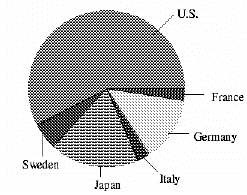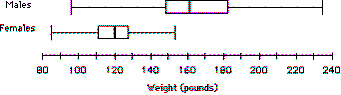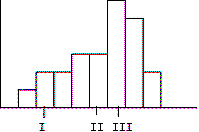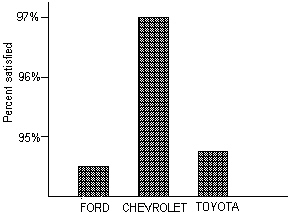Name:    AP Statistics Chapter 1 Test Study Guide

Multiple Choice
Identify the choice that best completes the statement or answers the question.

1.

You measure the age, marital status and earned income of an SRS of 1463 women.  The number and type of variables you have measured is
 a. 1463; all quantitative. b. four; two categorical and two quantitative. c. four; one categorical and three quantitative. d. three; two categorical and one quantitative. e. three; one categorical and two quantitative.

2.

Consumers’ Union measured the gas mileage in miles per gallon of 38 1978–1979 model automobiles on a special test track.  The pie chart below provides information about the country of manufacture of the model cars used by Consumers Union.  Based on the pie chart, we may conclude that:a. Japanese cars get significantly lower gas mileage than cars of other countries.  This is because their slice of the pie is at the bottom of the chart. b. U.S cars get significantly higher gas mileage than cars from other countries. c. Swedish cars get gas mileages that are between those of Japanese and U.S. cars. d. Mercedes, Audi, Porsche, and BMW represent approximately a quarter of the cars tested. e. More than half of the cars in the study were from the United States.

3.

“Normal” body temperature varies by time of day.  A series of readings was taken of the body temperature of a subject.  The mean reading was found to be 36.5° C with a standard deviation of 0.3° C (recall that °F = °C(1.8) + 32).  When converted to °F, the mean and standard deviation are:
 a. 97.7, 32 b. 97.7, 0.30 c. 97.7, 0.54 d. 97.7, 0.97 e. 97.7, 1.80

4.

Which of the following is likely to have a mean that is smaller than the median?
 a. The salaries of all National Football League players. b. The scores of students (out of 100 points) on a very easy exam in which most get nearly perfect scores but a few do very poorly. c. The prices of homes in a large city. d. The scores of students (out of 100 points) on a very difficult exam in which most get poor scores but a few do very well. e. Amounts awarded by civil court juries.

5.

The weights of the male and female students in a class are summarized in the following boxplots:Which of the following is NOT correct?
 a. About 50% of the male students have weights between 150 and 185 pounds. b. About 25% of female students have weights more than 130 pounds. c. The median weight of male students is about 162 pounds. d. The mean weight of female students is about 120 pounds because of symmetry. e. The male students have less variability than the female students.

6.

For the following histogram, what is the proper ordering of the mean, median, and mode?  Note that the graph is NOT numerically precise–only the relative positions are important.a. I = mean, II = median, III = mode b. I = mode, II = median, III = mean c. I = median, II = mean, III = mode d. I = mode, II = mean, III = median e. I = mean, II = mode, III = median

7.

A medical researcher collects health data on many women in each of several countries.  One of the variables measured for each woman in the study is her weight in pounds.  The following list gives the five-number summary for the weights of women in one of the countries.

Country A:      100, 110, 120, 160, 200

About what percentage of Country A women weigh between 110 and 200 pounds?
 a. 50% b. 65% c. 75% d. 85% e. 95%

8.

The following bar graph gives the percent of owners of three brands of trucks who are satisfied with their truck.From this graph we may legitimately conclude that:
 a. Owners of other brands of trucks are less satisfied than the owners of these three brands. b. Chevrolet owners are substantially more satisfied than Ford or Toyota owners. c. There is very little difference in the satisfaction of owners for the three brands. d. Chevrolet probably sells more trucks than Ford or Toyota. e. A pie chart would have been a better choice for displaying this data.

9.

A sample of 99 distances has a mean of 24 feet and a median of 24.5 feet.  Unfortunately, it has just been discovered that an observation which was erroneously recorded as “30” actually had a value of “35”.  If we make this correction to the data, then:
 a. The mean remains the same, but the median is increased. b. The mean and median remain the same. c. The median remains the same, but the mean is increased. d. The mean and median are both increased. e. We do not know how the mean and median are affected without further calculations, but the variance is increased.

10.

The five-number summary for scores on a statistics exam is 11, 35, 61, 70, 79.  In all, 380 students took the test.  About how many had scores between 35 and 61?
 a. 26 b. 76 c. 95 d. 190 e. None of these